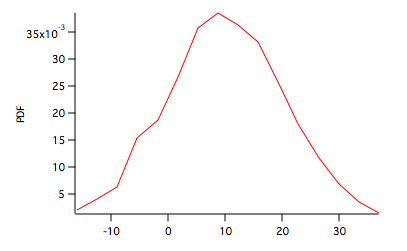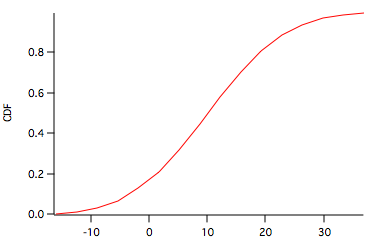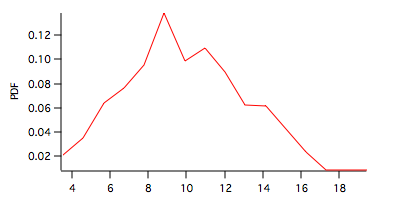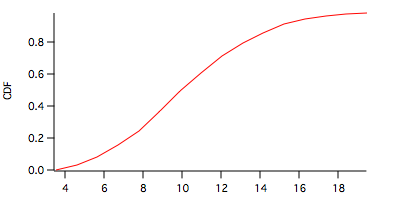The test provides a measure that indicates the fit of the distribution of your data to some known distribution. To illustrate how it works we start by generating 1000 pseudo-random data points with a Gaussian distribution:

`Make/N=1000 data1=10+gnoise(10)`

Although it is not necessary for the purpose of the test, we compute the cumulative distribution function for this data set using the following commands:

`Make/N=16/O W_Hist;DelayUpdate`
`Histogram/P/B=4 data1,W_Hist`
`Integrate/METH=1 W_Hist/D=W_INT`Here W_Hist is the PDF shown above and W_INT is the CDF shown below.To compare this to a normal CDF we can use the built-in StatsNormalCDF in the following user function:

Function getUserCDF(inX)

Variable inX

return statsNormalCDF(inX,10,10)

End

To run the KS test execute the command:

`StatsKSTest/T=1/CDFF=getUserCDF data1`

Note that the operation's input are the raw data and a user defined continuous distribution function. The results are shown in the Kolmogorov-Smirnov Test table:

 alpha 0.05 N 1000 D 0.0184245 Critical 0.0427766 PValue 0.501019

Since the test statistic D is smaller than the critical value we can't reject the null hypothesis that the two distributions are the same.

To illustrate a difference between distributions, suppose your data have a Poisson distribution as in:

`Make/n=1000 data2=poissonNoise(10)`Here the PDF is shown in the graph above and the CDF below.To test this data set against the same normal distribution as above you can execute the command:

```StatsKSTest/T=1/CDFF=getUserCDF data2

which yields the following table:

```
 alpha 0.05 N 1000 D 0.286538 Critical 0.0427766 PValue 1.80085e-73

In this case the test statistic D is clearly larger than the critical value and the hypothesis that data2 is taken from a normal distribution is rejected.ForumSupportGallery